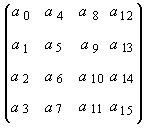# glMultMatrixf function

The glMultMatrixd and glMultMatrixf functions multiply the current matrix by an arbitrary matrix.

## Syntax

``````void WINAPI glMultMatrixf(
const GLfloat *m
);
``````

## Parameters

m

A pointer to a 4x4 matrix stored in column-major order as 16 consecutive values.

## Return value

This function does not return a value.

## Error codes

The following error code can be retrieved by the glGetError function.

Name Meaning
GL_INVALID_OPERATION
The function was called between a call to glBegin and the corresponding call to glEnd.

## Remarks

The glMultMatrix function multiplies the current matrix by the one specified in m. That is, if M is the current matrix and T is the matrix passed to glMultMatrix, then M is replaced with M T.

The current matrix is the projection matrix, modelview matrix, or texture matrix, determined by the current matrix mode (see glMatrixMode).

The m parameter points to a 4x4 matrix of single-precision or double-precision floating-point values stored in column-major order. That is, the matrix is stored as shown in the following image.The following functions retrieve information related to glMultMatrix:

glGet with argument GL_MATRIX_MODE

glGet with argument GL_MODELVIEW_MATRIX

glGet with argument GL_PROJECTION_MATRIX

glGet with argument GL_TEXTURE_MATRIX

## Requirements

Requirement Value
Minimum supported client
Windows 2000 Professional [desktop apps only]
Minimum supported server
Windows 2000 Server [desktop apps only]
Gl.h
Library
Opengl32.lib
DLL
Opengl32.dll